Select Page

# Regular Expressions and Finite Automata

Regular Expressions and Finite Automata

Contents

Describe Regular Expression, Operators, and Identities used in RE 1

Describe Precedence of Regular Expression Operator 5

State and apply Kleene’s Theorem and Arden’s Theorem 6

Design RE for the given Sets 7

Explain the Procedure to Eliminate Epsilon Move from FA 7

Explain Algebraic Law using Arden’s Theorem to find the Regular Expression for a given FA 7

Convert Finite Automata to Regular Expression 7

Explain the rules for designing a FA for the given RE 7

Design FA for the given Regular Expression 7

Explain the Equivalence of two Finite Automata 7

Explain the Equivalence of two Regular Expressions 7

Explain Chomsky Classification of Languages 7

Describe Type0, Type1, Type2, and Type3 Grammars 7

Identify Type0, Type1, Type2, and Type3 Grammars 7

Define Grammar 7

Generate Language for a given Grammar 7

Generate Grammar for the given Language 7

Describe Regular Grammar 7

Construct Regular Grammar for the given FA 7

Construct Regular Grammar for a given Regular Expression 7

Design FA for the given Regular Grammar 7

Define Pumping Lemma for Regular Language 7

Recall Applications of Pumping Lemma 7

Apply Pumping Lemma to prove that certain Sets are not Regular 7

Describe Union and Intersection Properties of Regular Languages 7

Describe Concatenation and Difference Properties of Regular Languages 7

Complementation and Homomorphism Properties of Regular Languages 7

# Describe Regular Expression, Operators, and Identities used in RE

Regular expressions are a concise and powerful notation for describing patterns in strings. They are widely used in text processing, pattern matching, and programming languages to search, manipulate, and validate strings. Regular expressions consist of various operators and identities that define the pattern matching rules.

Here’s a description of regular expressions, operators, and identities commonly used:

• Regular Expression:

A regular expression is a sequence of characters that defines a search pattern. It consists of literal characters and metacharacters that represent classes of characters or operations.

Operators in Regular Expressions:

1. Concatenation (AB): Concatenation is denoted by simply placing two regular expressions side by side. It matches the concatenation of strings that match the first expression followed by strings that match the second expression.
2. Alternation (A|B): Alternation allows for matching either of the expressions separated by the “|” (pipe) symbol. It matches strings that match either the left expression or the right expression.
3. Kleene Star (A*): The Kleene star denotes zero or more occurrences of the preceding regular expression. It matches strings that have zero or more repetitions of the expression.
4. Kleene Plus (A+): The Kleene plus denotes one or more occurrences of the preceding regular expression. It matches strings that have one or more repetitions of the expression.
5. Optional (A?): The optional operator “?” denotes zero or one occurrence of the preceding regular expression. It matches strings that have zero or one occurrence of the expression.
6. Grouping (()): Grouping allows grouping subexpressions together. It helps in defining the scope of operators and enables capturing groups for further use.

Identities in Regular Expressions:

1. Identity Element for Alternation: A|∅ = A

The identity element for alternation is the empty set (∅). When alternated with any regular expression, it yields the same regular expression.

1. Identity Element for Concatenation: A∅ = ∅A = ∅

The identity element for concatenation is the empty set (∅). When concatenated with any regular expression, it results in the empty set.

1. Identity Element for Kleene Star: A* = ε

The identity element for the Kleene star is the empty string (ε). The Kleene star applied to any regular expression gives the empty string.

1. Idempotent Property of Alternation: A|A = A

The alternation operator is idempotent, meaning that if the same regular expression is alternated with itself, it remains unchanged.

1. Identity Element for Concatenation with Empty String: Aε = εA = A

Concatenating any regular expression with the empty string (ε) results in the same regular expression.

1. Distributive Property of Concatenation over Alternation: A(B|C) = AB|AC

The distributive property allows distributing the concatenation operation over alternation. It means that concatenating a regular expression with an alternation of two expressions is equivalent to the alternation of the concatenation of the first expression with the second expression individually.

1. Absorption Property of Alternation with Empty String: A|ε = ε|A = A

The absorption property states that if a regular expression is alternated with the empty string (ε), the result is the same as the original regular expression.

1. Idempotent Property of Concatenation: A.A = A

Concatenating a regular expression with itself is equivalent to the original regular expression.

1. Identity Element for Kleene Plus: A+ = AA*

The Kleene plus operation on a regular expression is equivalent to concatenating the regular expression with its Kleene star.

1. Identity Element for Optional Operator: A? = A|ε

The optional operator on a regular expression is equivalent to alternation with the empty string (ε).

These operators and identities provide the foundation for constructing regular expressions and enable powerful pattern matching capabilities. By combining these operators and using literals and metacharacters, complex patterns can be described concisely and efficiently.

Regular Expression:

A regular expression is a sequence of characters that defines a search pattern. It is used for matching and manipulating strings based on specific patterns.

Example of a Regular Expression:

Pattern: “abc”

This regular expression matches the string “abc” exactly.

Operators in Regular Expressions:

1. Concatenation (AB):

Example:

Pattern: “ab”

This regular expression matches any string that has the characters “a” followed by “b”.

1. Alternation (A|B):

Example:

Pattern: “cat|dog”

This regular expression matches either the string “cat” or the string “dog”.

1. Kleene Star (A*):

Example:

Pattern: “a*”

This regular expression matches any string that contains zero or more occurrences of the character “a”.

1. Kleene Plus (A+):

Example:

Pattern: “a+”

This regular expression matches any string that contains one or more occurrences of the character “a”.

1. Optional (A?):

Example:

Pattern: “colou?r”

This regular expression matches either the string “color” or the string “colour”.

Identities in Regular Expressions:

1. Identity Element for Alternation:

Example:

Pattern: “A|∅”

This regular expression is equivalent to “A” since alternation with an empty set (∅) yields the original expression.

1. Identity Element for Concatenation:

Example:

Pattern: “Aε”

This regular expression is equivalent to “A” since concatenation with the empty string (ε) does not change the expression.

1. Identity Element for Kleene Star:

Example:

Pattern: “A*”

This regular expression matches any string that contains zero or more occurrences of the regular expression “A”. An empty string is also matched since the Kleene star can represent zero occurrences.

1. Idempotent Property of Alternation:

Example:

Pattern: “A|A”

This regular expression is equivalent to “A” since alternation with the same expression results in the original expression.

1. Identity Element for Concatenation with Empty String:

Pattern: “Aε”

This regular expression is equivalent to “A” since concatenating any regular expression with the empty string (ε) results in the same regular expression.

1. Distributive Property of Concatenation over Alternation:

Pattern: “A(B|C)”

This regular expression is equivalent to “AB|AC” since the concatenation of “A” with an alternation of “B” and “C” is the same as the alternation of the concatenation of “A” with “B” and the concatenation of “A” with “C”.

1. Absorption Property of Alternation with Empty String:

Pattern: “A|ε”

This regular expression is equivalent to “A” since alternation with the empty string (ε) yields the same regular expression.

1. Idempotent Property of Concatenation:

Pattern: “AA”

This regular expression is equivalent to “A” since concatenating a regular expression with itself is the same as the original regular expression.

1. Identity Element for Kleene Plus:

Pattern: “A+”

This regular expression matches any string that contains one or more occurrences of the regular expression “A”. It is equivalent to “AA*”, where “A*” represents zero or more occurrences.

1. Identity Element for Optional Operator:

Pattern: “A?”

This regular expression is equivalent to “A|ε”, as it matches either the regular expression “A” or the empty string (ε).

These examples illustrate the usage of regular expressions, operators, and identities to define patterns and match strings based on specific rules and conditions. Regular expressions provide a flexible and powerful way to manipulate and process textual data.

# Describe Precedence of Regular Expression Operator

The precedence of regular expression operators determines the order in which the operators are evaluated when constructing or matching a regular expression. It helps to define the grouping and hierarchy of operations within a regular expression.

The following is a typical precedence order for the common regular expression operators, listed from highest to lowest:

1. Grouping and Parentheses: ()

Grouping operators are evaluated first to specify the scope of operations within parentheses. They allow grouping subexpressions together and establish precedence within the expression.

1. Kleene Operators: *, +, ?

Kleene operators have higher precedence and are evaluated after grouping. They define the repetition of the preceding element or subexpression.

1. Concatenation: AB

Concatenation has higher precedence than alternation. It denotes the concatenation of two regular expressions or subexpressions.

1. Alternation: |

Alternation is evaluated last among the common operators. It represents the choice between two or more alternative regular expressions or subexpressions.

It’s important to note that although this is a general precedence order, parentheses can be used to override the default precedence and explicitly specify the desired evaluation order.

Example:

Consider the regular expression (ab+c)*d | ef+g

Here is how the precedence of operators is applied:

1. Parentheses: (ab+c)*d is evaluated as a subexpression, allowing the repetition of the sequence “ab+c” followed by the literal “d”.
2. Kleene Operator: (ab+c)* is evaluated, indicating zero or more occurrences of the subexpression “ab+c”.
3. Concatenation: (ab+c)d represents the concatenation of the subexpression (ab+c) with the literal “d”.
4. Alternation: (ab+c)*d | ef+g allows a choice between the subexpression (ab+c)*d and the subexpression ef+g.

By understanding the precedence of regular expression operators, you can construct and interpret regular expressions more accurately and ensure the intended matching behavior.

# State and apply Kleene’s Theorem and Arden’s Theorem

Kleene’s Theorem and Arden’s Theorem are important results in the theory of regular languages and finite automata.

• Kleene’s Theorem:

Kleene’s Theorem states that for any regular language L, there exists a regular expression that describes L. In other words, regular languages can be characterized by regular expressions.

• Application of Kleene’s Theorem:

To apply Kleene’s Theorem, we start with a given regular language and construct a corresponding regular expression using the following steps:

1. Start with the regular language L and represent it using its corresponding NFA or DFA.
2. Convert the NFA or DFA into a regular expression using methods such as the state elimination method, the Arden’s Theorem, or any other suitable technique.
3. The resulting regular expression represents the original regular language L.
• Arden’s Theorem:

Arden’s Theorem provides a method to find a unique solution for a system of linear equations involving regular expressions. It states that given two regular expressions A and B, the equation X = AX + B has a unique solution X, which is also a regular expression.

• Application of Arden’s Theorem:

To apply Arden’s Theorem, we follow these steps:

1. Given a system of linear equations involving regular expressions, set up the equations in the form X = AX + B, where A and B are regular expressions.
2. Apply Arden’s Lemma, which states that for regular expressions A and B, the equation X = AX + B has a unique solution X = A* B.
3. Solve the equation using the Arden’s Lemma to obtain the regular expression X, which represents the solution to the system of equations.

By applying Arden’s Theorem, we can find the solution to systems of linear equations involving regular expressions, which can be useful in various applications such as compiler design, pattern matching, and string manipulation.

Both Kleene’s Theorem and Arden’s Theorem provide important theoretical foundations for working with regular languages and regular expressions, allowing us to describe and manipulate these languages effectively.

Proof of Arden’s Theorem:

To prove Arden’s Theorem, we need to show that for any regular expressions A and B, the equation X = AX + B has a unique solution X = A* B.

Proof of Arden’s Theorem:

Step 1: Construct the Regular Expression X = A* B.

We start by assuming X = A* B and prove that it satisfies the equation X = AX + B.

Step 2: Show that X = AX + B.

We can rewrite the equation X = AX + B as X – AX = B.

Using the distributive property of concatenation over alternation, we can rewrite it as X(I – A) = B, where I is the identity element for concatenation.

Step 3: Prove that X = A* B is a solution to the equation.

To prove this, we need to show that X = A* B satisfies the equation X(I – A) = B.

Consider the expression X(I – A). Since X = A* B, we substitute it into the equation:

X(I – A) = (A* B)(I – A).

Using the identity element for concatenation, we have:

(A* B)(I – A) = A* B – A(A* B).

Now, we apply the distributive property of concatenation over alternation to the second term:

A* B – A(A* B) = A* B – A(A*)B.

Using the identity element for concatenation, A* A = A*, we simplify further:

A* B – A(A*)B = A* B – A* B.

This simplifies to:

A* B – A* B = 0.

Therefore, we have shown that X = A* B satisfies the equation X(I – A) = B.

Step 4: Show uniqueness of the solution.

To prove uniqueness, assume there exists another regular expression Y that satisfies the equation X(I – A) = B.

Let’s assume Y is a different solution such that Y = AY + B.

Substituting X = A* B and Y = AY + B into the equation, we have:

A* B(I – A) = B.

By simplifying the equation, we get:

A* B – A* BA = B.

Using the distributive property and the identity element for concatenation, we have:

A* B – A* BA = A* B – A* B = 0.

Therefore, Y = A* B is the unique solution to the equation X = AX + B.

Hence, we have proved Arden’s Theorem, which states that for any regular expressions A and B, the equation X = AX + B has a unique solution X = A* B.

# Design RE for the given Sets

Here are 10 examples of regular expressions for different sets, listed from lower to higher difficulty:

1. Set: {a}

Regular Expression: a

Description: Matches the single character ‘a’.

1. Set: {a, b}

Regular Expression: a|b

Description: Matches either the character ‘a’ or ‘b’.

1. Set: {ab, ac}

Regular Expression: a[b|c]

Description: Matches the string ‘ab’ or ‘ac’.

1. Set: {a, aa, aaa}

Regular Expression: a{1,3}

Description: Matches the strings ‘a’, ‘aa’, or ‘aaa’.

1. Set: {0, 10, 100, 1000}

Regular Expression: 10{0,3}

Description: Matches the strings ‘0’, ’10’, ‘100’, or ‘1000’.

1. Set: {a, ab, abb, abbb}

Regular Expression: ab{1,3}

Description: Matches the strings ‘a’, ‘ab’, ‘abb’, or ‘abbb’.

1. Set: {aa, aba, abba, abbba}

Regular Expression: (a(ba){0,3})

Description: Matches the strings ‘aa’, ‘aba’, ‘abba’, or ‘abbba’.

Regular Expression: a(b|d)c

Description: Matches the strings ‘abc’, ‘acb’, or ‘adc’.

1. Set: {ab, abb, abbb, abbbb}

Regular Expression: ab{1,}

Description: Matches the strings ‘ab’, ‘abb’, ‘abbb’, or ‘abbbb’. Allows one or more occurrences of ‘b’.

1. Set: {ab, aabb, aaabbb, aaaabbbb}

Regular Expression: a{1,}b{2,}

Description: Matches the strings ‘ab’, ‘aabb’, ‘aaabbb’, or ‘aaaabbbb’. Allows one or more occurrences of ‘a’ followed by two or more occurrences of ‘b’.

These examples gradually increase in difficulty, introducing more complex patterns and quantifiers to match different sets of strings.

Here aresome examples of finding regular expressions for different languages or strings:

1. Language: Set of all strings over {a, b} that start with ‘aa’:

Regular Expression: aa(a|b)*

Description: Matches any string that starts with ‘aa’, allowing any number of ‘a’ or ‘b’ characters after ‘aa’.

1. Language: Set of all strings over {0, 1} that have an odd number of ‘0’ characters:

Regular Expression: (1(01)*0)*1

Description: Matches any string that has an odd number of ‘0’ characters, allowing any number of alternating ‘1’ and ’01’ sequences before the last ‘1’.

1. Language: Set of all strings over {a, b} that contain at least one ‘ab’ or ‘ba’ substring:

Regular Expression: (a|b)((ab)|(ba))(a|b)

Description: Matches any string that contains at least one occurrence of ‘ab’ or ‘ba’, allowing any number of ‘a’ or ‘b’ characters before, in between, and after.

1. Language: Set of all strings over {a, b} that end with ‘aa’:

Regular Expression: (a|b)*(aa)

Description: Matches any string that ends with ‘aa’, allowing any number of ‘a’ or ‘b’ characters before ‘aa’.

# Explain the Procedure to Eliminate Epsilon Move from FA

The procedure to eliminate epsilon (ε) moves from a finite automaton (FA) involves several steps. Here is a general procedure to follow:

1. Identify epsilon closure: Compute the epsilon closure for each state in the FA. The epsilon closure of a state is the set of all states that can be reached from it by following epsilon transitions.
2. Create a new DFA: Create a new deterministic finite automaton (DFA) that will replace the original FA without epsilon moves.
3. Initialize the new DFA: Start by adding the epsilon closure of the initial state of the original FA as the initial state of the new DFA.
4. Explore transitions: For each state in the new DFA, explore transitions on input symbols other than epsilon. Determine the set of states that can be reached from the current state by following transitions on each input symbol.
5. Add transitions: Add transitions to the new DFA based on the sets of states reached in the previous step. Each set of states becomes a new state in the DFA, and transitions are added accordingly.
6. Finalize states: Identify the final states of the new DFA. A state in the new DFA is a final state if it contains any final state of the original FA.
7. Repeat steps 4-6: Repeat steps 4 to 6 for each new state added to the DFA until no more new states can be added.
8. The resulting DFA: The resulting DFA obtained after eliminating epsilon moves will have no epsilon transitions, and it recognizes the same language as the original FA.

It is important to note that the procedure to eliminate epsilon moves can be complex for more intricate FAs. In some cases, it may involve constructing and analyzing an epsilon-NFA and then converting it to a DFA.

# Explain Algebraic Law using Arden’s Theorem to find the Regular Expression for a given FA

The two commonly used methods for converting a given DFA to its regular expression are:

1. Arden’s Method
2. State Elimination Method

Arden’s Theorem is a popular technique employed to convert a given DFA to its corresponding regular expression. The theorem states the following:

Let P and Q be two regular expressions over ∑.

If P does not contain the empty string ε, then the equation R = Q + RP has a unique solution, which is R = QP*.

To use Arden’s Theorem, certain conditions must be satisfied:

1. The transition diagram should not include any ε transitions.
2. There should be only a single initial state.

The process of converting a DFA to its regular expression using Arden’s Theorem involves the following steps:

Step 1:

Formulate an equation for each state, considering the transitions leading to that state. Include the empty string ε in the equation of the initial state.

Step 2:

Manipulate the equations to bring the final state into the form R = Q + RP, which yields the desired regular expression.

Important notes to consider:

• Arden’s Theorem can be applied to both DFAs and NFAs.
• If there are multiple final states, create a separate regular expression for each one and combine them by adding all the regular expressions to obtain the final regular expression.

# Convert Finite Automata to Regular Expression

Example 1: Find regular expression for the following DFA using Arden’s Theorem-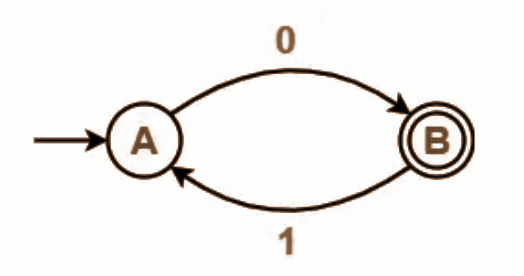Form a equation for each state-

• A = ∈ + B.1 ……(1)
• B = A.0 ……(2)

Bring final state in the form R = Q + RP.

Using (1) in (2), we get-

B = (∈ + B.1).0

B = ∈.0 + B.1.0

B = 0 + B.(1.0) ……(3)

Using Arden’s Theorem in (3), we get-

B = 0.(1.0)*

Thus, Regular Expression for the given DFA = 0(10)*

Example 2: Find regular expression for the following DFA using Arden’s Theorem-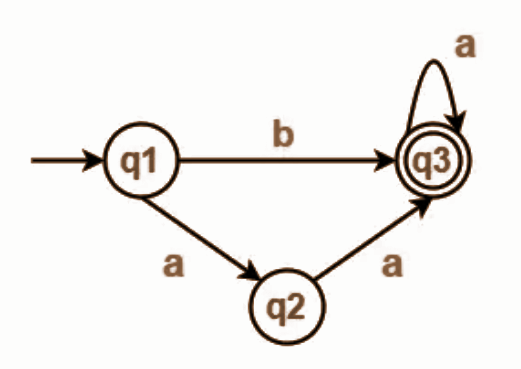Form a equation for each state-

• q1 = ∈ ……(1)
• q2 = q1.a ……(2)
• q3 = q1.b + q2.a + q3.a …….(3)

Bring final state in the form R = Q + RP.

Using (1) in (2), we get-

q2 = ∈.a

q2 = a …….(4)

Using (1) and (4) in (3), we get-

q3 = q1.b + q2.a + q3.a

q3 = ∈.b + a.a + q3.a

q3 = (b + a.a) + q3.a …….(5)

Using Arden’s Theorem in (5), we get-

q3 = (b + a.a)a*

Thus, Regular Expression for the given DFA = (b + aa)a*

Example 3: Find regular expression for the following DFA using Arden’s Theorem-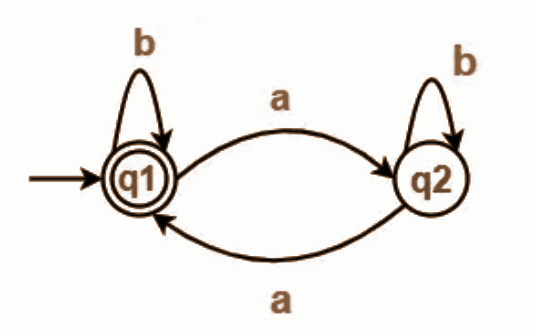Form a equation for each state-

• q1 = ∈ + q1.b + q2.a ……(1)
• q2 = q1.a + q2.b ……(2)

Bring final state in the form R = Q + RP.

Using Arden’s Theorem in (2), we get-

q2 = q1.a.b* …….(3)

Using (3) in (1), we get-

q1 = ∈ + q1.b + q1.a.b*.a

q1 = ∈ + q1.(b + a.b*.a) …….(4)

Using Arden’s Theorem in (4), we get-

q1 = ∈.(b + a.b*.a)*

q1 = (b + a.b*.a)*

Thus, Regular Expression for the given DFA = (b + a.b*.a)*

# Explain the rules for designing a FA for the given RE

When designing a finite automaton (FA) for a given regular expression, there are certain rules and guidelines to follow. The purpose of designing an FA is to construct a machine that recognizes the language defined by the regular expression.

Here are the rules for designing an FA based on a regular expression:

• If the regular expression is ε (empty string), design an FA with a single state that is also a final state.
• If the regular expression is a single symbol, design an FA with two states connected by a transition on that symbol. The initial state is not a final state, and the destination state is a final state.
2. Rule 2: Handle concatenation (concatenation operator):
• For a regular expression R1R2 (concatenation of R1 and R2), design an FA for R1 and an FA for R2.
• Make the final state(s) of the FA for R1 non-final.
• Connect the final state(s) of the FA for R1 to the initial state(s) of the FA for R2 with epsilon transitions.
• The initial state of the FA for R1 becomes the initial state of the resulting FA, and the final state(s) of the FA for R2 become the final state(s) of the resulting FA.
3. Rule 3: Handle union (OR operator):
• For a regular expression R1 + R2 (union of R1 and R2), design an FA for R1 and an FA for R2.
• Create a new initial state and connect it to the initial states of the FAs for R1 and R2 with epsilon transitions.
• Create a new final state and make the final states of the FAs for R1 and R2 non-final, connecting them to the new final state with epsilon transitions.
• The new initial state becomes the initial state of the resulting FA, and the new final state becomes the final state of the resulting FA.
4. Rule 4: Handle Kleene star (closure operator):
• For a regular expression R*, design an FA for R.
• Create a new initial state and a new final state.
• Connect the new initial state to the initial state of the FA for R and to the new final state with epsilon transitions.
• Make the final states of the FA for R non-final, connecting them to the initial state of the FA for R and to the new final state with epsilon transitions.
• The new initial state becomes the initial state of the resulting FA, and the new final state becomes the final state of the resulting FA.

By applying these rules recursively, you can design an FA for any given regular expression. The resulting FA will recognize the language defined by the regular expression.

# Design FA for the given Regular Expression

Example 1: Design a FA from given regular expression 10 + (0 + 11)0* 1.

Solution: First we will construct the transition diagram for a given regular expression.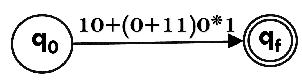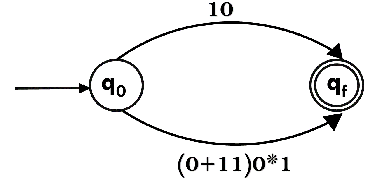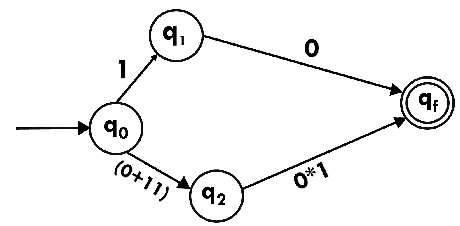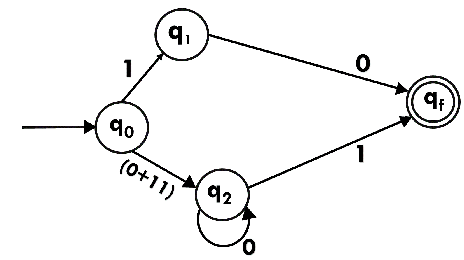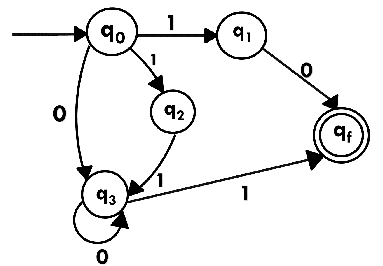Now we have got NFA without ε. Now we will convert it into required DFA for that, we will first write a transition table for this NFA.

 State 0 1 →q0 q3 {q1, q2} q1 qf ϕ q2 ϕ q3 q3 q3 qf *qf ϕ ϕ

The equivalent DFA will be:

 State 0 1 →[q0] [q3] [q1, q2] [q1] [qf] ϕ [q2] ϕ [q3] [q3] [q3] [qf] [q1, q2] [qf] [qf] *[qf] ϕ ϕ

Example 2: Design a NFA from given regular expression 1 (1* 01* 01*)*.

Solution: The NFA for the given regular expression is as follows: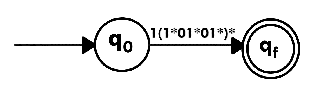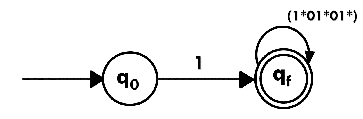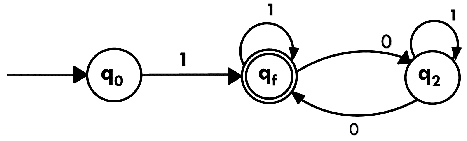Example 3: Construct the FA for regular expression 0*1 + 10.

Solution:

We will first construct FA for R = 0*1 + 10 as follows: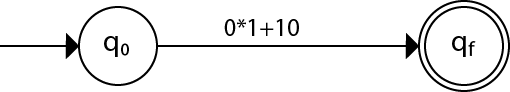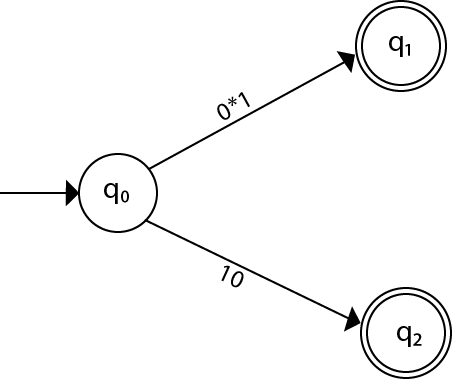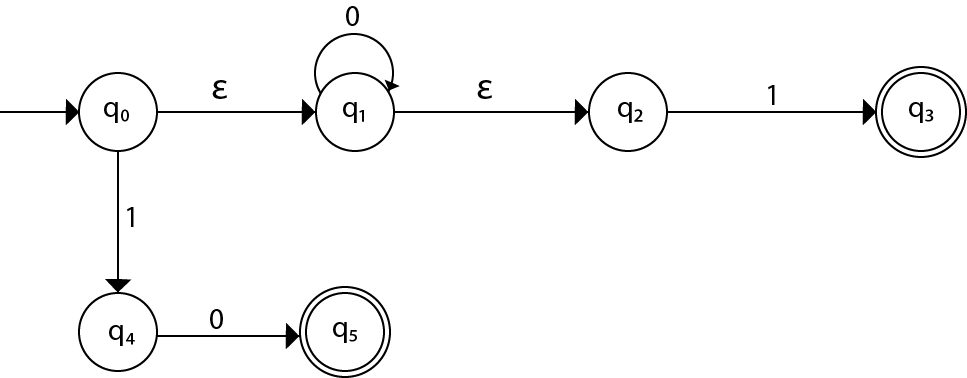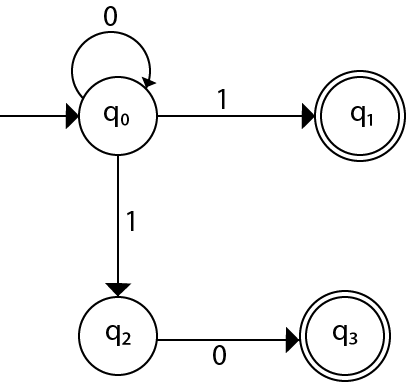# Explain the Equivalence of two Finite Automata

The equivalence of two finite automata (FA) refers to the concept that both automata recognize the same language. In other words, if two FAs produce the same outputs for the same inputs, they are considered equivalent.

To determine the equivalence of two FAs, we need to compare their behaviors and transitions. Here are the steps to establish the equivalence between two FAs:

1. States: Verify that both FAs have the same set of states. If they have a different number of states or if the states are not matching, the FAs are not equivalent.
2. Alphabet: Check if both FAs have the same alphabet, which is the set of input symbols or characters that the automata can process. If the alphabets differ, the FAs cannot be equivalent.
3. Initial State: Verify that both FAs have the same initial state. The initial state is the state where the automaton starts its computation. If the initial states differ, the FAs are not equivalent.
4. Transitions: Examine the transition function of both FAs. The transition function defines how the automaton moves from one state to another based on the input symbol. Compare the transitions for each state and input symbol in both FAs. If the transitions differ for any state-input combination, the FAs are not equivalent.
5. Final States: Check if both FAs have the same set of final states, also known as accepting states or end states. These states indicate that the automaton has reached an accepting condition for a given input sequence. If the final states differ, the FAs are not equivalent.

If all the above conditions are satisfied, the two FAs are considered equivalent as they recognize the same language. It means that both automata produce the same outputs for all possible inputs.

Determining the equivalence of FAs is crucial in various applications, such as language recognition, pattern matching, and optimization of automata.

Let’s consider an example to demonstrate the equivalence of two finite automata (FAs):

FA1:

• States: q0, q1, q2
• Alphabet: {0, 1}
• Initial State: q0
• Transitions:
• q0 on input 0 goes to q1
• q0 on input 1 goes to q0
• q1 on input 0 goes to q2
• q1 on input 1 goes to q0
• q2 on input 0 goes to q2
• q2 on input 1 goes to q2
• Final State: q2

FA2:

• States: A, B, C
• Alphabet: {0, 1}
• Initial State: A
• Transitions:
• A on input 0 goes to B
• A on input 1 goes to A
• B on input 0 goes to C
• B on input 1 goes to A
• C on input 0 goes to C
• C on input 1 goes to C
• Final State: C

To establish the equivalence between FA1 and FA2, we need to compare their behaviors and transitions:

1. States: Both FAs have the same set of states: {q0, q1, q2} = {A, B, C}.
2. Alphabet: Both FAs have the same alphabet: {0, 1}.
3. Initial State: Both FAs have the same initial state: q0 = A.
4. Transitions: Comparing the transitions for each state and input symbol, we find that the transitions in FA1 and FA2 are identical:
• For input 0:
• q0 goes to q1, which is equivalent to A goes to B.
• q1 goes to q2, which is equivalent to B goes to C.
• q2 goes to q2, which is equivalent to C goes to C.
• For input 1:
• q0 goes to q0, which is equivalent to A goes to A.
• q1 goes to q0, which is equivalent to B goes to A.
• q2 goes to q2, which is equivalent to C goes to C.
1. Final States: Both FAs have the same final state: q2 = C.

Since all the conditions for equivalence are satisfied, we can conclude that FA1 and FA2 are equivalent. Both FAs recognize the same language and produce the same outputs for all possible inputs.

# Explain the Equivalence of two Regular Expressions

The equivalence of two regular expressions refers to the concept that both expressions represent the same language. In other words, if two regular expressions generate the same set of strings or patterns, they are considered equivalent.

To establish the equivalence of two regular expressions, we need to compare their patterns and behaviors. Here are the steps to determine the equivalence between two regular expressions:

1. Patterns: Analyze the patterns represented by both regular expressions. Consider the combinations of symbols, operators, and quantifiers used in each expression.
2. Language Recognition: Determine the languages recognized by each regular expression. A language is a set of strings that can be generated by the regular expression.
3. String Matching: Examine the strings that match each regular expression. Compare the sets of strings generated by each expression. If the sets of matching strings are the same, the regular expressions are equivalent.
4. Simplification: Simplify both regular expressions, if possible, using the laws and properties of regular expressions. This simplification process can help in comparing the patterns and revealing their equivalence.
5. Boolean Operations: Apply boolean operations such as union (|), concatenation, and closure (*) to the regular expressions. Observe if the resulting expressions are equivalent or can be transformed into each other.

If, after performing the above steps, it is determined that the patterns, languages, and matching strings of both regular expressions are the same, then the regular expressions are considered equivalent.

Establishing the equivalence of regular expressions is important in various applications, such as pattern matching, string manipulation, and language processing. By recognizing the equivalence, we can determine that two expressions can be used interchangeably to represent the same language or set of strings.

Let’s consider an example to demonstrate the equivalence of two regular expressions:

Regular Expression 1: (a|b)*

Regular Expression 2: (b|a)*

To establish the equivalence between the two regular expressions, we need to compare their patterns and behaviors:

1. Patterns: Both regular expressions have the same pattern of (a|b)*, where either ‘a’ or ‘b’ can occur zero or more times.
2. Language Recognition: Both regular expressions recognize the same language, which is the set of all possible strings consisting of ‘a’ and ‘b’, including the empty string.
3. String Matching: Both regular expressions generate the same set of matching strings, including ‘a’, ‘b’, ‘aa’, ‘ab’, ‘ba’, ‘bb’, ‘aaa’, ‘aab’, ‘aba’, ‘abb’, and so on. The set of matching strings is identical for both regular expressions.
4. Simplification: Both regular expressions are already in their simplest form, and no further simplification can be applied.
5. Boolean Operations: If we apply the union (|) operator to Regular Expression 1 or Regular Expression 2, the resulting expression is (a|b)*, which is equivalent to both original expressions.

Based on the comparison of patterns, language recognition, matching strings, and simplification, we can conclude that Regular Expression 1 and Regular Expression 2 are equivalent. Both expressions represent the same language, generate the same set of matching strings, and can be used interchangeably to describe the same pattern.

# Explain Chomsky Classification of Languages

Chomsky’s classification of languages, also known as the Chomsky hierarchy, is a framework proposed by linguist Noam Chomsky to categorize formal languages based on their generative power and the types of grammar used to describe them.

The Chomsky hierarchy consists of four types of languages: Type-3, Type-2, Type-1, and Type-0, arranged in increasing order of generative power.

1. Type-3: Regular Languages (Regular Expressions and Finite Automata)
• Regular languages are the simplest and most restricted type of formal languages.
• They can be described by regular expressions or recognized by finite automata.
• Regular languages are characterized by their regular grammars, which consist of rules with simple patterns and limited expressive power.
• Regular languages can be used to describe patterns that can be processed efficiently using finite automata.
2. Type-2: Context-Free Languages (Context-Free Grammars and Pushdown Automata)
• Context-free languages are more expressive than regular languages.
• They can be described by context-free grammars or recognized by pushdown automata.
• Context-free grammars have rules with a single non-terminal symbol on the left-hand side and a sequence of terminals and non-terminals on the right-hand side.
• Context-free languages are widely used in programming languages, syntax analysis, and natural language processing.
3. Type-1: Context-Sensitive Languages (Context-Sensitive Grammars and Linear Bounded Automata)
• Context-sensitive languages have even greater expressive power than context-free languages.
• They can be described by context-sensitive grammars or recognized by linear bounded automata.
• Context-sensitive grammars allow rules where the left-hand side and right-hand side can have different lengths and contain both terminals and non-terminals.
• Context-sensitive languages are used in complex syntactic and semantic analysis tasks.
4. Type-0: Recursively Enumerable Languages (Unrestricted Grammars and Turing Machines)
• Recursively enumerable languages have the highest generative power and flexibility.
• They can be described by unrestricted grammars or recognized by Turing machines.
• Unrestricted grammars have rules with no restrictions on the left-hand side and right-hand side.
• Recursively enumerable languages can describe any computable language and can recognize any language that can be recognized by a Turing machine.

The Chomsky hierarchy provides a systematic classification of formal languages based on their generative power and the types of grammars needed to describe them. Each type of language is associated with a specific class of grammars and automata, which have different levels of expressive power and computational complexity. The hierarchy is an essential tool in formal language theory and helps in understanding the limitations and capabilities of different types of languages.

# Describe Type0, Type1, Type2, and Type3 Grammars

Here are the descriptions of Type-0, Type-1, Type-2, and Type-3 grammars, along with examples:

1. Type-0: Unrestricted Grammars
• Type-0 grammars, also known as unrestricted grammars or phrase structure grammars, have the most generative power among all grammars.
• In Type-0 grammars, there are no restrictions on the form of production rules.
• The left-hand side of a production rule can be any string of symbols, and the right-hand side can be any string of symbols, including both terminals and non-terminals.
• These grammars are associated with recursively enumerable languages.
• Example: S → aSb
2. Type-1: Context-Sensitive Grammars
• Type-1 grammars have a more restricted form than Type-0 grammars.
• In Type-1 grammars, the left-hand side of a production rule can be expanded to a string of symbols that is no longer than the right-hand side.
• The context-sensitive grammars have the form αAβ → αγβ, where A is a non-terminal, α and β are strings of symbols (including empty string), and γ is a string of symbols.
• These grammars are associated with context-sensitive languages.
• Example: ABC → BC
3. Type-2: Context-Free Grammars
• Type-2 grammars, also known as context-free grammars, have a simpler form than Type-1 grammars.
• In Type-2 grammars, the left-hand side of a production rule consists of a single non-terminal symbol.
• The context-free grammars have the form A → α, where A is a non-terminal and α is a string of symbols.
• These grammars are associated with context-free languages.
• Example: S → aSb
4. Type-3: Regular Grammars
• Type-3 grammars, also known as regular grammars, are the simplest and most restricted form of grammars.
• In Type-3 grammars, the left-hand side of a production rule consists of a single non-terminal symbol.
• The right-hand side of a production rule can be either a single terminal symbol, or a terminal symbol followed by a non-terminal symbol.
• These grammars are associated with regular languages.
• Example: A → a, A→ aB

It’s important to note that each type of grammar is a proper subset of the grammars in the higher levels of the Chomsky hierarchy. In other words, the generative power of grammars increases from Type-3 to Type-0, with Type-0 grammars being the most powerful.

# Identify Type0, Type1, Type2, and Type3 Grammars

Here are the descriptions of Type-0, Type-1, Type-2, and Type-3 grammars, along with examples:

1. Type-0: Unrestricted Grammars
• Type-0 grammars, also known as unrestricted grammars or phrase structure grammars, have the most generative power among all grammars.
• In Type-0 grammars, there are no restrictions on the form of production rules.
• The left-hand side of a production rule can be any string of symbols, and the right-hand side can be any string of symbols, including both terminals and non-terminals.
• These grammars are associated with recursively enumerable languages.
• Example: S → aSb
2. Type-1: Context-Sensitive Grammars
• Type-1 grammars have a more restricted form than Type-0 grammars.
• In Type-1 grammars, the left-hand side of a production rule can be expanded to a string of symbols that is no longer than the right-hand side.
• The context-sensitive grammars have the form αAβ → αγβ, where A is a non-terminal, α and β are strings of symbols (including empty string), and γ is a string of symbols.
• These grammars are associated with context-sensitive languages.
• Example: ABC → BC
3. Type-2: Context-Free Grammars
• Type-2 grammars, also known as context-free grammars, have a simpler form than Type-1 grammars.
• In Type-2 grammars, the left-hand side of a production rule consists of a single non-terminal symbol.
• The context-free grammars have the form A → α, where A is a non-terminal and α is a string of symbols.
• These grammars are associated with context-free languages.
• Example: S → aSb
4. Type-3: Regular Grammars
• Type-3 grammars, also known as regular grammars, are the simplest and most restricted form of grammars.
• In Type-3 grammars, the left-hand side of a production rule consists of a single non-terminal symbol.
• The right-hand side of a production rule can be either a single terminal symbol, or a terminal symbol followed by a non-terminal symbol.
• These grammars are associated with regular languages.
• Example: A → a

It’s important to note that each type of grammar is a proper subset of the grammars in the higher levels of the Chomsky hierarchy. In other words, the generative power of grammars increases from Type-3 to Type-0, with Type-0 grammars being the most powerful.

# Define Grammar

In the theory of computation, a grammar is a formal system that describes the syntax and structure of a language. It is used to generate valid strings or sentences of the language and to define the rules for constructing those strings.

A grammar consists of a set of production rules that define how symbols or characters in the language can be combined or rearranged to form valid strings. These production rules specify the syntactic patterns or patterns of construction for the language.

Formally, a grammar is defined by a 4-tuple (N, Σ, P, S), where:

• N is a set of non-terminal symbols or variables that represent syntactic categories or parts of speech.
• Σ is a set of terminal symbols or alphabet that represent the basic elements or tokens of the language.
• P is a set of production rules that specify how to rewrite non-terminal symbols into a sequence of terminal and/or non-terminal symbols.
• S is the start symbol that represents the initial non-terminal symbol from which the language derivation starts.

The process of deriving or generating valid strings from a grammar is typically done by applying the production rules repeatedly, starting with the start symbol, until only terminal symbols remain. The resulting strings are valid sentences of the language defined by the grammar.

Grammars play a fundamental role in formal language theory and are used to study the properties and structures of various types of languages, such as regular languages, context-free languages, and more complex languages. They are an essential tool in the analysis and design of programming languages, compilers, parsers, and natural language processing systems.

# Generate Language for a given Grammar

To generate a language for a given grammar, we follow the process of applying production rules starting from the start symbol until only terminal symbols remain.

Here’s a step-by-step procedure to generate a language from a grammar:

2. Apply a production rule by replacing a non-terminal symbol with its corresponding production.
3. Repeat the process for each non-terminal symbol in the generated string until only terminal symbols remain.
4. Continue applying production rules until all possible derivations are exhausted.

Let’s consider an example to illustrate the process:

Grammar:

S → aSb

S → ε

Language to generate: {a^n b^n | n ≥ 0}

Step 2: Apply the first production rule S → aSb, replacing S with aSb.

Result: aSb

Step 3: Apply the first production rule S → aSb again, replacing S with aSb.

Result: aaSbb

Step 4: Apply the second production rule S → ε, replacing S with ε.

Result: aabb

Step 5: Stop because no further non-terminal symbols are present.

The language generated by this grammar is {ε, ab, aabb, aaabbb, …}, which represents the set of strings of the form anb, where n is greater than or equal to 0.

Note that the number of iterations determines the length of the generated strings. In this example, we can generate strings of any length by applying the first production rule multiple times. The ε production rule allows for the generation of an empty string as well.

By following the production rules and applying them appropriately, we can generate various languages based on the grammar’s structure and restrictions.

# Generate Grammar for the given Language

To generate a grammar for a given language, we need to analyze the patterns and rules that define the language. Here’s a step-by-step procedure to generate a grammar for a given language:

1. Identify the language’s patterns and rules by examining its elements and desired structure.
2. Define the set of non-terminal symbols (variables) that represent different syntactic categories or parts of speech in the language.
3. Define the set of terminal symbols (alphabet) that represent the basic elements or tokens of the language.
4. Formulate the production rules that define how the non-terminal symbols can be combined or rearranged to form valid strings in the language. These rules should adhere to the patterns and restrictions of the language.
5. Specify the start symbol that represents the initial non-terminal symbol from which the language derivation starts.

Let’s consider an example to illustrate the process:

Language: {w | w contains an even number of ‘a’s and an odd number of ‘b’s}

Step 1: Analyze the language:

• The language consists of strings with an even number of ‘a’s and an odd number of ‘b’s.

Step 2: Define non-terminal and terminal symbols:

• Non-terminal symbols: S, A, B
• Terminal symbols: a, b

Step 3: Formulate production rules:

• S → AB | A
• A → aaA | ε
• B → bBb | b

Step 4: Specify the start symbol:

• Start symbol: S

The resulting grammar for the given language is:

S → AB | A

A → aaA | ε

B → bBb | b

This grammar generates strings such as ‘bbb’, ‘aabbb’, ‘aaaabbbbb’, ‘aaabbb’, and so on, where the number of ‘a’s is even and the number of ‘b’s is odd.

# Describe Regular Grammar

A regular grammar, also known as a right-linear grammar or a type-3 grammar, is a formal grammar that generates regular languages. Regular grammars are defined by a set of production rules that have specific restrictions on their form.

Here are the characteristics of a regular grammar:

1. Non-terminal symbols: A regular grammar has a finite set of non-terminal symbols, which are variables that represent different syntactic categories or parts of speech.
2. Terminal symbols: A regular grammar has a finite set of terminal symbols, which are the basic elements or tokens of the language. Terminal symbols are the characters or symbols that appear in the generated strings.
3. Production rules: The production rules of a regular grammar have the following form:
• A → aB
• A → a
• A → ε
4. In these rules:
• A and B are non-terminal symbols.
• a is a terminal symbol.
• ε represents the empty string.
5. The production rules can be read as “A can be replaced by aB”, “A can be replaced by a”, or “A can be replaced by ε”. The first two types of rules involve one non-terminal symbol followed by a terminal symbol or a non-terminal symbol. The last type of rule allows for the generation of the empty string.
6. Start symbol: A regular grammar has a designated start symbol, which is a non-terminal symbol that represents the initial symbol from which the language derivation starts.

Regular grammars are used to generate regular languages, which are a class of languages that can be recognized by finite automata such as deterministic finite automata (DFA) or non-deterministic finite automata (NFA). Regular languages are characterized by their linear structure and can be described by regular expressions.

Regular grammars are less expressive compared to other types of grammars, such as context-free grammars or context-sensitive grammars, but they are useful for describing simple patterns and regular languages encountered in many applications, including lexical analysis, pattern matching, and regular expression matching.

# Construct Regular Grammar for the given FA

To construct a regular grammar for a given FA, we need to follow these steps:

1. Identify the start state of the FA and make it the start symbol of the grammar.
2. For each transition in the FA, create a production of the form A → aB or A → a, where A and B are non-terminal symbols and a is a terminal symbol.
3. For each final state in the FA, create a production of the form A → ε, where A is the corresponding non-terminal symbol.

Alternately, To construct a regular grammar for a given finite automaton (FA), we need to follow these steps:

1. Analyze the FA:
• Identify the set of states (Q).
• Identify the input alphabet (Σ).
• Identify the transition function (δ).
• Identify the initial state (q0).
• Identify the set of final states (F).
2. Define non-terminal and terminal symbols:
• Each state in the FA will correspond to a non-terminal symbol in the regular grammar.
• Each symbol in the input alphabet will correspond to a terminal symbol in the regular grammar.
3. Formulate production rules:
• For each transition in the FA, create a production rule in the regular grammar.
• The production rule will have the form A → aB, where A and B are non-terminal symbols representing states, and a is a terminal symbol representing an input symbol.
4. Specify the start symbol:
• The start symbol of the regular grammar will correspond to the initial state of the FA.
5. Specify the set of final states:
• The non-terminal symbols corresponding to the final states in the FA will be designated as final symbols in the regular grammar.

Let’s consider an example to illustrate the construction of a regular grammar for a given FA:

FA:

• States (Q): q0, q1
• Input alphabet (Σ): {a, b}
• Transition function (δ):
• δ(q0, a) = q1
• δ(q1, b) = q0
• Initial state (q0): q0
• Final state (F): q1

Regular Grammar:

• Non-terminal symbols: S, A, B
• Terminal symbols: a, b
• Production rules:
• S → aA
• A → bS
• A → ε
• B → aB
• B → bA
• Start symbol: S
• Final symbols: A

The regular grammar generated for the given FA is:

S → aA

A → bS | ε

B → aB | bA

This grammar will generate strings that follow the same language as the given FA. In this case, it will generate strings where each ‘a’ is followed by a ‘b’ and vice versa, ending with an odd number of ‘b’s.

Please note that the example provided is for illustration purposes, and the construction of a regular grammar may vary depending on the specific FA.

# Construct Regular Grammar for a given Regular Expression

To construct a regular grammar for a given regular expression, we need to follow these steps:

1. Analyze the regular expression:
• Identify the symbols in the regular expression.
• Determine the structure and operations involved, such as concatenation, union, and Kleene star.
2. Define non-terminal and terminal symbols:
• Each operation or subexpression in the regular expression will correspond to a non-terminal symbol in the regular grammar.
• Each symbol in the regular expression will correspond to a terminal symbol in the regular grammar.
3. Formulate production rules:
• Based on the operations and subexpressions in the regular expression, create production rules in the regular grammar.
• The production rules will mirror the structure and operations of the regular expression.
4. Specify the start symbol:
• The start symbol of the regular grammar can be assigned to the non-terminal symbol corresponding to the entire regular expression.
5. Specify the acceptance condition:
• Determine the acceptance condition for the regular grammar, such as specifying a final non-terminal symbol or a production rule that generates the empty string.

Let’s consider an example to illustrate the construction of a regular grammar for a given regular expression:

Let’s correct the regular grammar for the regular expression a+:

Regular Expression: a+

Regular Grammar:

• Non-terminal symbol: S
• Terminal symbol: a
• Production rules:
• S → aS | a

Explanation:

• The non-terminal symbol S represents the start symbol of the grammar.
• The terminal symbol a represents the input symbol.
• The production rule S → aS allows the repetition of ‘a’ zero or more times, generating strings with one or more occurrences of ‘a’.
• The production rule S → a generates a string with a single ‘a’.

The regular grammar generated for the regular expression a+ is:

S → aS | a

This grammar will generate strings consisting of one or more occurrences of ‘a’.

Please note that the example provided is for the specific regular expression a+. The construction of a regular grammar may vary depending on the given regular expression.

# Design FA for the given Regular Grammar

To design an FA for a given regular grammar, we can follow these steps:

1. Identify the start symbol of the regular grammar and make it the start state of the FA.
2. For each production of the form A → aB, create a transition from state A to state B on input symbol a.
3. For each production of the form A → a, create a transition from state A to a final state on input symbol a.
4. If there is a production of the form S → ε, make the start state and any final states reachable from the start state as final states.

Alternately, To design a Finite Automaton (FA) for a given Regular Grammar, we need to follow these steps:

1. Identify the non-terminal symbols and terminal symbols of the grammar.
2. Determine the start symbol and any final symbols of the grammar.
3. Construct the states of the FA based on the non-terminal symbols of the grammar.
4. Define the transitions between states based on the terminal symbols and production rules of the grammar.
5. Mark the start state and any final states in the FA.

Let’s illustrate this process with an example:

Regular Grammar:

• Non-terminal symbol: S
• Terminal symbols: a, b
• Production rules:
• S → aS | b

Finite Automaton:

States: {S, F}

Transitions:

• (S, a) → S
• (S, b) → F

Start state: S

Final state: F

In this corrected example, the FA has two states, S and F. The transition (S, a) indicates that when the input is ‘a’, the FA remains in state S. The transition (S, b) leads to the final state F. There are no other transitions defined for the final state F. The start state is S, and the final state is F.

# Define Pumping Lemma for Regular Language

The Pumping Lemma for Regular Languages is a fundamental result in the theory of computation that helps prove that a language is not regular. It provides a criterion to show that a language is not regular by demonstrating that it cannot satisfy the pumping property.

The Pumping Lemma states the following:

For every regular language L, there exists a constant ‘p’ (the pumping length) such that for any string ‘s’ in L with length at least ‘p’, we can split ‘s’ into three parts, ‘xyz’, satisfying the following conditions:

1. The middle part ‘y’ is not empty, i.e., y ≠ ε.
2. The length of the string ‘xy’ is at most ‘p’.
3. For any non-negative integer ‘n’, the string ‘xyⁿz’ (concatenation of ‘x’, ‘y’ repeated ‘n’ times, and ‘z’) is also in L.

In other words, the Pumping Lemma guarantees that if a language is regular, then every sufficiently long string in that language can be split into parts in such a way that repeating the middle part any number of times while keeping the other parts intact will still result in a string within the language.

By using the Pumping Lemma, if it can be shown that a language L does not satisfy the conditions outlined above for any choice of ‘p’, then it can be concluded that L is not a regular language.

It is important to note that the Pumping Lemma can only prove that a language is not regular, but it cannot prove that a language is regular. Regular languages will satisfy the conditions of the Pumping Lemma, but not all languages that fail the Pumping Lemma are necessarily non-regular.

# Recall Applications of Pumping Lemma

The Pumping Lemma for Regular Languages is a powerful tool in the theory of computation that allows us to demonstrate the non-regularity of certain languages. Although it cannot prove that a language is regular, it is widely used to identify languages that are not regular.

Here are some applications of the Pumping Lemma:

1. Proving the non-regularity of a language: The Pumping Lemma can be applied to show that a language is not regular. By assuming the language is regular and demonstrating a contradiction using the Pumping Lemma, we can conclude that the language is not regular.
2. Providing evidence for non-regularity: Even if the Pumping Lemma cannot conclusively prove that a language is not regular, it can provide strong evidence for non-regularity. If we are able to find a counterexample that violates the conditions of the Pumping Lemma, it strongly suggests that the language is not regular.
3. Guiding the design of non-regular languages: The Pumping Lemma can be used as a guiding principle to construct non-regular languages. By ensuring that the language violates the conditions of the Pumping Lemma, we can create languages that are not regular.
4. Enhancing our understanding of regular languages: Even when we are not specifically aiming to prove non-regularity, applying the Pumping Lemma can provide insights into the structure and limitations of regular languages. It helps us understand the inherent constraints and properties of regular languages.

It is important to note that while the Pumping Lemma is a valuable tool, it has its limitations. There are non-regular languages that satisfy the conditions of the Pumping Lemma, and not all languages that fail the Pumping Lemma are necessarily non-regular. Therefore, the Pumping Lemma should be used judiciously in combination with other techniques to determine the regularity of languages.

# Apply Pumping Lemma to prove that certain Sets are not Regular

The Pumping Lemma is often used to prove that certain sets or languages are not regular. Let’s go through the general process of applying the Pumping Lemma to prove non-regularity:

1. Assume the language is regular: Begin by assuming that the language in question is regular.
2. Choose a specific string: Select a specific string from the language that satisfies the conditions of the Pumping Lemma. The string should have a length greater than or equal to the pumping length ‘p’ associated with the language.
3. Split the string: Divide the selected string into three parts: ‘xyz’, where ‘y’ is the part that can be pumped.
4. Verify the conditions: Check if the conditions of the Pumping Lemma hold for the chosen string:

a. The length of ‘xy’ is at most ‘p’.

b. ‘y’ is not empty (y ≠ ε).

c. For any non-negative integer ‘n’, the string ‘xyⁿz’ is also in the language.

1. Show a contradiction: Demonstrate that at least one of the conditions fails to hold. This indicates that the language cannot be regular, contradicting the initial assumption.

By successfully showing a contradiction, it can be concluded that the language is not regular.

It’s important to note that the Pumping Lemma is a sufficient condition for non-regularity but not a necessary condition. That means if the conditions of the Pumping Lemma are not violated, it does not guarantee that the language is regular. However, if the conditions are violated, it provides strong evidence that the language is not regular.

Here are three examples demonstrating the application of the Pumping Lemma to prove non-regularity:

Example 1: Language L = {0^n1^n | n ≥ 0}

Assume L is regular.

Choose the string s = 0^p1^p, where p is the pumping length.

Split s into three parts: x = ε, y = 0^p, z = 1^p.

By pumping y, the resulting string becomes xy^2z = 0^p0^p1^p, which is not in L since the number of 0s and 1s are not equal.

This violates the condition that for any non-negative integer n, the string xy^nz must still be in L.

Thus, L is not regular.

Example 2: Language L = {ww | w is a string of 0s and 1s}

Assume L is regular.

Choose the string s = 0^p1^p0^p1^p, where p is the pumping length.

Split s into three parts: x = 0^p, y = 1^p, z = 0^p1^p.

By pumping y, the resulting string becomes xy^2z = 0^p1^p1^p0^p1^p, which is not in L since the first and second halves are not equal.

This violates the condition that for any non-negative integer n, the string xy^nz must still be in L.

Therefore, L is not regular.

Example 3: Language L = {a^n b^n c^n | n ≥ 0}

Assume L is regular.

Choose the string s = a^pb^pc^p, where p is the pumping length.

Split s into three parts: x = a^p, y = b^p, z = c^p.

By pumping y, the resulting string becomes xy^2z = a^pb^pc^pb^pc^p, which is not in L since the number of b’s and c’s are not equal.

This violates the condition that for any non-negative integer n, the string xy^nz must still be in L.

Thus, L is not regular.

In each example, we assumed that the language is regular and then chose a specific string that satisfies the conditions of the Pumping Lemma.

By pumping a specific part of the string, we obtained a string that does not belong to the language, violating the conditions of the Pumping Lemma. Therefore, we concluded that the languages are not regular.

# Describe Union and Intersection Properties of Regular Languages

The union and intersection of regular languages are also regular languages.

The union of two regular languages L1 and L2, denoted by L1 ∪ L2, is the language that contains all strings that are either in L1 or in L2 or in both. In other words,

L1 ∪ L2 = {w | w ∈ L1 or w ∈ L2}.

The intersection of two regular languages L1 and L2, denoted by L1 ∩ L2, is the language that contains all strings that are in both L1 and L2. In other words,

L1 ∩ L2 = {w | w ∈ L1 and w ∈ L2}.

To prove that the union and intersection of regular languages are regular, we can use either of the following methods:

1. Construct a DFA for the union/intersection: Given DFAs for L1 and L2, we can construct a DFA for L1 ∪ L2 or L1 ∩ L2 by taking the Cartesian product of the two DFAs and defining the transitions accordingly.
2. Use regular expressions: Given regular expressions for L1 and L2, we can use the regular expression operators ∪ and ∩ to obtain a regular expression for L1 ∪ L2 or L1 ∩ L2.

Therefore, the union and intersection of regular languages are regular languages.

Alternately, The union and intersection properties are important properties of regular languages. Let’s explore each property with examples:

1. Union Property:

The union property states that the union of two regular languages is also a regular language. In other words, if L1 and L2 are regular languages, then their union L1 ∪ L2 is also a regular language.

Example:

Consider two regular languages:

L1 = {a^n | n ≥ 0} (language of strings with zero or more ‘a’)

L2 = {b^n | n ≥ 0} (language of strings with zero or more ‘b’)

The union of L1 and L2, denoted by L1 ∪ L2, is the language that contains all strings that belong to either L1 or L2 (or both).

L1 ∪ L2 = {ε, a, aa, …, b, bb, …}

The union property tells us that the resulting language L1 ∪ L2 is also regular.

1. Intersection Property:

Let’s consider a corrected example for the intersection property of regular languages:

Consider two regular languages:

L1 = {a^n | n ≥ 0} (language of strings with one or more ‘a’s)

L2 = {b^n | n ≥ 0} (language of strings with one or more ‘b’s)

The intersection of L1 and L2, denoted by L1 ∩ L2, is the language that contains all strings that belong to both L1 and L2.

L1 ∩ L2 = ∅ (empty language)

In this example, the resulting language L1 ∩ L2 is the empty language. Since L1 consists of strings with one or more ‘a’s and L2 consists of strings with one or more ‘b’s, there are no strings that satisfy both conditions simultaneously.

The intersection property states that if the resulting language L1 ∩ L2 is empty, it is still considered a regular language, as the empty language can be recognized by a finite automaton.

# Describe Concatenation and Difference Properties of Regular Languages

The concatenation and difference of regular languages may not necessarily result in a regular language.

1. The concatenation of two regular languages L1 and L2, denoted by L1L2, is the language that contains all strings that can be formed by concatenating a string from L1 and a string from L2. In other words, L1L2 = {xy | x ∈ L1 and y ∈ L2}.

To prove that the concatenation of two regular languages is regular, we can use the following method:

Construct a non-deterministic finite automaton (NFA) for the concatenation: Given an NFA for L1 and an NFA for L2, we can construct an NFA for L1L2 by adding ε-transitions from the accepting states of the NFA for L1 to the start state of the NFA for L2.

1. The difference between two regular languages L1 and L2, denoted by L1-L2, is the language that contains all strings that are in L1 but not in L2. In other words, L1-L2 = {w | w ∈ L1 and w ∉ L2}.

Alternately,

Concatenation Property of Regular Languages:

The concatenation property of regular languages states that if L1 and L2 are regular languages, then their concatenation, denoted by L1L2, is also a regular language. The concatenation of two languages is formed by concatenating every string in L1 with every string in L2.

For example, let L1 = {a, aa} and L2 = {b, bb}. Their concatenation L1L2 is {ab, abb, aab, aabb}. Each string in L1L2 is formed by concatenating a string from L1 with a string from L2.

The concatenation property is a fundamental property of regular languages and can be proved using various methods such as finite automata, regular expressions, or regular grammar.

Difference Property of Regular Languages:

The difference property of regular languages states that if L1 and L2 are regular languages, then their difference, denoted by L1 – L2, is also a regular language. The difference of two languages represents all the strings that belong to L1 but do not belong to L2.

For example, let L1 = {a, b, c, d} and L2 = {b, d}. Their difference L1 – L2 is {a, c}. It includes all the strings from L1 that are not present in L2.

The difference property can be proved by constructing a finite automaton or regular expression that recognizes the desired difference language.

Both the concatenation and difference properties demonstrate that regular languages are closed under these operations, meaning that applying these operations to regular languages will always result in another regular language. These properties are important in the study of formal languages and automata theory.

# Complementation and Homomorphism Properties of Regular Languages

The complement of a regular language L, denoted by L’, is the language that contains all strings over the alphabet of L that are not in L. In other words, L’ = {w | w is over the alphabet of L and w ∉ L}.

To prove that the complement of a regular language is regular, we can use the following method:

1. Construct a deterministic finite automaton (DFA) for L: We can use the subset construction algorithm to convert an NFA for L into an equivalent DFA.
2. Reverse the final and non-final states of the DFA: This results in a new DFA that accepts the complement of L.

The homomorphic image of a regular language L with respect to a homomorphism h is the language that contains all strings that can be obtained by applying h to each symbol of a string in L. In other words, h(L) = {h(w) | w ∈ L}.

To prove that the homomorphic image of a regular language is regular, we can use the following method:

1. Construct a DFA for L: We can use the subset construction algorithm to convert an NFA for L into an equivalent DFA.
2. Construct a new DFA for h(L): For each symbol in the alphabet of the original DFA, replace it with its corresponding image under the homomorphism h. This results in a new DFA that accepts h(L).

Therefore, the complementation and homomorphism of regular languages result in regular languages.

Alternately,

Complementation Property of Regular Languages:

The complementation property of regular languages states that if L is a regular language, then its complement, denoted by L’, is also a regular language. The complement of a language represents all the strings that do not belong to the original language.

For example, let L be the language of all strings over the alphabet {a, b} that start with an ‘a’: L = {a, ab, aaa, abb, …}. The complement of L, denoted by L’, will contain all strings over the same alphabet that do not start with an ‘a’: L’ = {ε, b, bb, bab, …}.

The complementation property can be proved by constructing a finite automaton or regular expression that recognizes the complement language. It involves inverting the accepting and non-accepting states of the original automaton or changing the regular expression to represent the complement language.

Homomorphism Property of Regular Languages:

The homomorphism property of regular languages states that if L is a regular language over the alphabet Σ, and h is a homomorphism from Σ to Σ’, then the language obtained by applying the homomorphism h to L, denoted by h(L), is also a regular language over the alphabet Σ’.

A homomorphism is a mapping that preserves the structure of strings. It maps each symbol of the original alphabet to a string of symbols in the new alphabet. The homomorphism is then applied to each string in the original language, resulting in a language over the new alphabet.

For example, let L be the language over the alphabet {a, b} defined as L = {ab, ba}. Suppose we have a homomorphism h defined as h(a) = X and h(b) = Y, where X and Y are symbols in the new alphabet {X, Y}. Applying the homomorphism to L, we get h(L) = {XY, YX}, which is a language over the alphabet {X, Y}.

The homomorphism property can be proved by constructing a finite automaton or regular expression that recognizes the language h(L) using the transformed alphabet.

Both the complementation and homomorphism properties demonstrate that regular languages are closed under these operations, meaning that applying these operations to regular languages will always result in another regular language. These properties play a crucial role in the study of formal languages and automata theory.